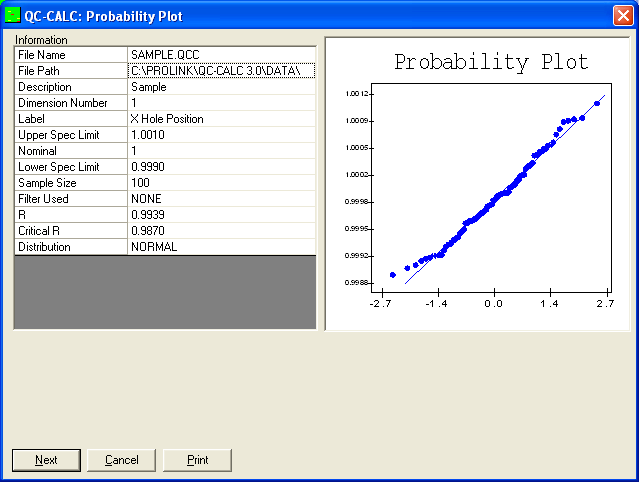###### Probability Plot
Probability Plots

The Normal Probability Plot chart provides a test for normality that is more sensitive than the Chi Squared test when the sample is small. This test works with sample sizes as small as 3, and works well with sample sizes between 20 and 100.

This chart is equivalent to plotting the data on normal probability paper, drawing a straight (Normal) line on the graph with the same mean and standard deviation as the sample, drawing vertical lines through each data point, and calculating the correlation coefficient, R, between the data points and the corresponding points on the Normal line. The process is considered normal if the correlation coefficient is sufficiently close to 1, with the critical value determined by consulting the table printed in the manual. The significance level is .05, giving a 5% chance of rejecting data from a normal process.